# 11.E: Exercises

•• Anonymous
• LibreTexts
$$\newcommand{\vecs}{\overset { \rightharpoonup} {\mathbf{#1}} }$$ $$\newcommand{\vecd}{\overset{-\!-\!\rightharpoonup}{\vphantom{a}\smash {#1}}}$$$$\newcommand{\id}{\mathrm{id}}$$ $$\newcommand{\Span}{\mathrm{span}}$$ $$\newcommand{\kernel}{\mathrm{null}\,}$$ $$\newcommand{\range}{\mathrm{range}\,}$$ $$\newcommand{\RealPart}{\mathrm{Re}}$$ $$\newcommand{\ImaginaryPart}{\mathrm{Im}}$$ $$\newcommand{\Argument}{\mathrm{Arg}}$$ $$\newcommand{\norm}{\| #1 \|}$$ $$\newcommand{\inner}{\langle #1, #2 \rangle}$$ $$\newcommand{\Span}{\mathrm{span}}$$ $$\newcommand{\id}{\mathrm{id}}$$ $$\newcommand{\Span}{\mathrm{span}}$$ $$\newcommand{\kernel}{\mathrm{null}\,}$$ $$\newcommand{\range}{\mathrm{range}\,}$$ $$\newcommand{\RealPart}{\mathrm{Re}}$$ $$\newcommand{\ImaginaryPart}{\mathrm{Im}}$$ $$\newcommand{\Argument}{\mathrm{Arg}}$$ $$\newcommand{\norm}{\| #1 \|}$$ $$\newcommand{\inner}{\langle #1, #2 \rangle}$$ $$\newcommand{\Span}{\mathrm{span}}$$

#### 11.2:  Representing Valence Electrons with Dots

1. Is it necessary for the first dot around an atomic symbol to go on a particular side of the atomic symbol?

No. The first dot can go on any side of the atomic symbol.

1. What column of the periodic table has Lewis electron dot diagrams with two electrons?

IIA or 2

1. What column of the periodic table has Lewis electron dot diagrams that have six electrons in them?

VIA or 16

1. Draw the Lewis electron dot diagram for each element.
1. strontium
2. silicon
1. $$⋅\,\mathrm{Sr}\,⋅$$
2.  $$\overset⬝{\underset⬝{⋅\,\mathrm{Si}\,⋅}}$$

1. Draw the Lewis electron dot diagram for each element.
1. krypton
2. sulfur
1. $$\overset{⬝\,⬝}{\underset{⬝\,⬝}{:\mathrm{Kr}:}}$$
2. $$\overset⬝{\underset{⬝\,⬝}{:\mathrm{S}\,⋅}}$$

1. Draw the Lewis electron dot diagram for each element.
1. potassium
2. phosphorus
1. $$\mathrm K\,⋅$$
2. $$\overset⬝{\underset⬝{:\mathrm{P}\,⋅}}$$

1. Draw the Lewis electron dot diagram for each element.
1. bromine
2. gallium
1. $$\overset{⬝\,⬝}{\underset{⬝\,⬝}{:\mathrm{Br}\,⋅}}$$
2. $$\overset⬝{\underset⬝{\mathrm{Ga}}}\,⋅$$

#### 11.3:  Ionic Bonds

1. How many electrons does a Ba atom have to lose to have a complete octet in its valence shell?

2

1. How many electrons does a Pb atom have to lose to have a complete octet in its valence shell?

4

1. How many electrons does an Se atom have to gain to have a complete octet in its valence shell?

2

1. How many electrons does an N atom have to gain to have a complete octet in its valence shell?

3

1. Which of the following atoms would be expected to form negative ions in binary ionic compounds and which would be expected to form positive ions: P, I, Mg, Cl, In, Cs, O, Pb, Co?

P, I, Cl, and O would form anions because they are nonmetals. Mg, In, Cs, Pb, and Co would form cations because they are metals.

1. Which of the following atoms would be expected to form negative ions in binary ionic compounds and which would be expected to form positive ions: Br, Ca, Na, N, F, Al, Sn, S, Cd?

Br, N, F, and S would form anions because they are nonmetals. Ca, Na, Al, Sn, and Cd would form cations because they are metals.

1. Predict the charge on the monatomic ions formed from the following atoms in binary ionic compounds:
1. P
2. Mg
3. Al
4. O
5. Cl
6. Cs
1. P3-
2. Mg2+
3. Al3+
4. O2-
5. Cl-
6. Cs+

1. Predict the charge on the monatomic ions formed from the following atoms in binary ionic compounds:
1. I
2. Sr
3. K
4. N
5. S
6. Li
1. I-
2. Sr2+
3. K+
4. N3-
5. S2-
6. Li+

1. Name two ions with the same Lewis electron dot diagram as the Cl ion.

S2- and P3-

1. Draw the Lewis electron dot diagram for each ion.
1. Mg2+
2. S2−
1. Mg2+ (no electrons)
2. $$\left[\overset{⬝\,⬝}{\underset{⬝\,⬝}{:\mathrm{S}:}}\right]^{2-}$$

1. Draw the Lewis electron dot diagram for each ion.
1. H+
2. Br
1. H+ (no electrons)
2. $$\left[\overset{⬝\,⬝}{\underset{⬝\,⬝}{:\mathrm{Br}:}}\right]^-$$

1. Draw the Lewis electron dot diagram for each ion.
1. Al3+
2. N3−
1. Al3+ (no electrons)
2. $$\left[\overset{⬝\,⬝}{\underset{⬝\,⬝}{:\mathrm{N}:}}\right]^{3-}$$

1. Write the Lewis symbols for each of the following ions:
1. As3
2. I
3. Be2+
4. O2–
5. Ga3+
6. Li+
7. N3–
1. $$\left[\overset{⬝\,⬝}{\underset{⬝\,⬝}{:\mathrm{As}:}}\right]^{3-}$$
2. $$\left[\overset{⬝\,⬝}{\underset{⬝\,⬝}{:\mathrm{I}:}}\right]^-$$
3. Be2+ (no electrons)
4. $$\left[\overset{⬝\,⬝}{\underset{⬝\,⬝}{:\mathrm{O}:}}\right]^{2-}$$
5. Ga3+ (no electrons)
6. Li+ (no electrons)
7. $$\left[\overset{⬝\,⬝}{\underset{⬝\,⬝}{:\mathrm{N}:}}\right]^{3-}$$

1. Many monatomic ions are found in seawater, including the ions formed from the following list of elements. Write the Lewis symbols for the monatomic ions formed from the following elements:
1. Cl
2. Na
3. Mg
4. Ca
5. K
6. Br
7. Sr
8. F
1. $$\left[\overset{⬝\,⬝}{\underset{⬝\,⬝}{:\mathrm{Cl}:}}\right]^-$$
2. Na+ (no electrons)
3. Mg2+ (no electrons)
4. Ca2+ (no electrons)
5. K+ (no electrons)
6. $$\left[\overset{⬝\,⬝}{\underset{⬝\,⬝}{:\mathrm{Br}:}}\right]^-$$
7. Sr2+ (no electrons)
8. $$\left[\overset{⬝\,⬝}{\underset{⬝\,⬝}{:\mathrm{F}:}}\right]^-$$

#### 11.4:  Covalent Bonds

1. How many electrons will be in the valence shell of H atoms when it makes a covalent bond?

2

1. How many electrons will be in the valence shell of non-H atoms when they make covalent bonds?

8

1. What is the Lewis electron dot diagram of I21. What is the Lewis electron dot diagram of H2S?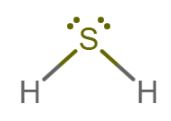1. What is the Lewis electron dot diagram of NCl3?1. What is the Lewis electron dot diagram of SiF4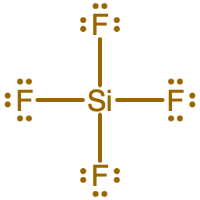#### 11.6:  Writing Lewis Structures for Molecular Compounds

1. Draw the Lewis structure for each substance.
1. SF2
2. C2Cl2 (assume two central atoms)
1.2.1. Draw the Lewis structure for each substance.
1. PI3
2. CS2
1.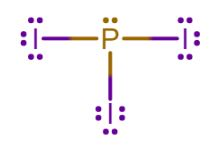2.1. Draw the Lewis structure for each substance.
1. GeH4
2. ClF
1.2.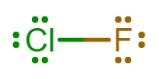1. Draw the Lewis structure for each substance.
1. AsF3
2. POCl
1.2.1. Draw the Lewis structure for each substance. Double or triple bonds may be needed.
1. SiO2
2. C2H4 (assume two central atoms)
1.2.1. Write the Lewis structure for the diatomic molecule P2, an unstable form of phosphorus found in high-temperature phosphorus vapor.1. Write Lewis structures for the following:
1. H2
2. HBr
3. PCl3
4. HNNH
5. H2CNH
1.2.3.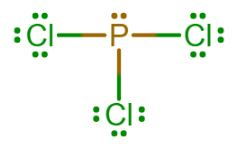4.5.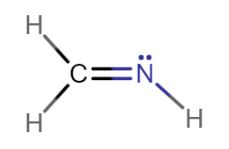1. Write Lewis structures for the following:
1. O2
2. H2CO
3. ClNO
4. SiCl4
5. ClCN
1.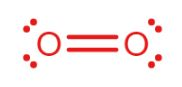2.3.4.5.1. Carbon tetrachloride was formerly used in fire extinguishers for electrical fires. It is no longer used for this purpose because of the formation of the toxic gas phosgene, Cl2CO. Write the Lewis structures for carbon tetrachloride and phosgene.

CCl4Cl2CO: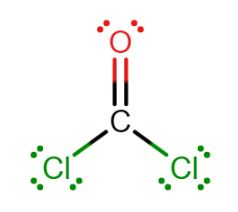1. The arrangement of atoms in several biologically important molecules is given here. Complete the Lewis structures of these molecules by adding multiple bonds and lone pairs. Do not add any more atoms.
1. the amino acid serine:2. urea:3. pyruvic acid:4. uracil:5. carbonic acid:1.2.3.4.5.#### 11.7:  Predicting the Shapes of Molecules

1. What is the basic premise behind VSEPR?

Electrons groups (bonds and lone pair electrons) want to be as far from each other as possible due to the negative charges in the groups repelling each other.

1. What is the difference between the electron group geometry and the molecular geometry?

Electron group geometry tells where all electron groups are located. Molecular geometry tells where the atoms are located.

1. Identify whether the following molecules are linear or bent.
1. H2S
2. HCN
3. CS2
1. bent
2. linear
3. linear

1. Identify whether the following molecules are linear or bent around the central atoms.
1. C2Cl2 (both C atoms are central atoms and are bonded to each other)
2. N2H2 (both N atoms are central atoms and are bonded to each other)